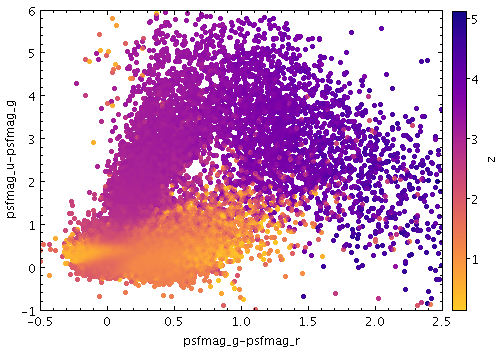Next Previous Up Contents
Next: Output Modes
Previous: aux

#### 8.4.7 `weighted`

Paints markers like the Density mode, but with optional weighting by an additional coordinate. You can configure how the weighted coordinates are combined to give the final weighted result. The way that data values are mapped to colours is usually controlled by options at the level of the plot itself, rather than by per-layer configuration.

Usage:

```   shadingN=weighted weightN=<num-expr> colorN=<rrggbb>|red|blue|...
combineN=sum|count|mean|median|...
```

All the parameters listed here affect only the relevant layer, identified by the suffix `N`.

Example:```   stilts plot2plane layer1=mark in1=dr5qso.fits
x1=psfmag_g-psfmag_r y1=psfmag_u-psfmag_g size1=2
xmin=-0.5 xmax=2.5 ymin=-1 ymax=6```

Associated parameters are as follows:

`colorN = <rrggbb>|red|blue|...`       (Color)
The color of plotted data, given by name or as a hexadecimal RGB value.

The standard plotting colour names are `red`, `blue`, `green`, `grey`, `magenta`, `cyan`, `orange`, `pink`, `yellow`, `black`, `light_grey`, `white`. However, many other common colour names (too many to list here) are also understood. The list currently contains those colour names understood by most web browsers, from `AliceBlue` to `YellowGreen`, listed e.g. in the Extended color keywords section of the CSS3 standard.

Alternatively, a six-digit hexadecimal number RRGGBB may be supplied, optionally prefixed by "`#`" or "`0x`", giving red, green and blue intensities, e.g. "`ff00ff`", "`#ff00ff`" or "`0xff00ff`" for magenta.

[Default: `red`]

`combineN = sum|count|mean|median|...`       (Combiner)
Defines how values contributing to the same pixel are combined together to produce the value assigned to that pixel (and hence its colour).

When a weight is in use, `mean` or `sum` are typically sensible choices. If there is no weight (a pure density map) then `count` is usually better, but in that case it may make more sense (it is more efficient) to use one of the other shading modes instead.

The available options are:

• `sum`: the sum of all the combined values per bin
• `count`: the number of non-blank values per bin (weight is ignored)
• `mean`: the mean of the combined values
• `median`: the median
• `q1`: first quartile
• `q3`: third quartile
• `min`: the minimum of all the combined values
• `max`: the maximum of all the combined values
• `stdev`: the sample standard deviation of the combined values
• `stdev_pop`: the population standard deviation of the combined values
• `hit`: 1 if any values present, NaN otherwise (weight is ignored)

[Default: `mean`]

`weightN = <num-expr>`       (String)
Weight coordinate for weighted density shading.

This parameter gives a column name, fixed value, or algebraic expression for the `weight` coordinate for layer `N`. The value is a numeric algebraic expression based on column names as described in Section 10.

Next Previous Up Contents
Next: Output Modes